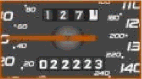## Odometers

An odometer measures the distance that a vehicle travels. Odometers, in recently built automobiles, measure up to a value of 999,999 kilometers. At 1,000,000 the odometer would restart from zero. This is referred to as odometer rollover.It is not uncommon to see an odometer displaying the same digit more than once. For example in the photo above, the digit 2 appears four times on the odometer that measures 022223. The next odometer reading that would contain the digit 3 four times would be 023333. This would occur 1110 kilometers later.

Your job as a computer programmer is to determine the distance in kilometers that would need to be travelled in order to find an odometer reading with a digit that has the same number of occurrences as another digit on another odometer reading.

The input will contain five sets of data. Each set of data will contain three lines. The first line will contain six digits that represent an odometer reading, R1. The second line will contain a single digit, d1, the digit whose number of occurences, N1, need to be determined. 2 ≤ N1 ≤ 6. The third line will contain a single digit, d2, the digit which will appear exactly N1 times on the next possible odometer reading, R2. d1 ≠ d2.

The output should contain five lines of data, corresponding to each set in the input. It will contain the next possible odometer reading on which the single digit, d2, will appear exactly N1 times and the number of kilometers to travel from R1 to R2. These two numbers are separated by a single space.

### Sample Input (Only three sets of data given)

```022223
2
3
010201
0
2
999969
9
6```

### Output

```023333 1110
010222 21
066666 66697```

Point Value: 7
Time Limit: 1.00s
Memory Limit: 32M

Languages Allowed:
C++03, PAS, C, HASK, ASM, RUBY, PYTH2, JAVA, PHP, SCM, CAML, PERL, C#, C++11, PYTH3